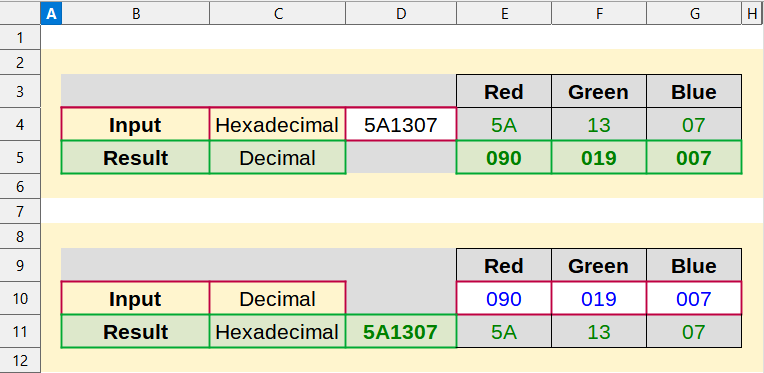# Convert color values

Other languages:
Deutsch • ‎English

## ForewordColor Value Conversion "Hexadecimal to Decimal" and "Decimal to Hexdecimal"

This description refers to the version LibreOffice 7.0 under Windows 10.

The Work file can be used immediately as a finished solution.

Link to the Video (in German [DE]):

## Convert color code

The color code RGB values (Red, Green, Blue) are often given in hexadecimal or decimal.

The hexadecimal code is 6-digits, 2-digits each for Red, Green and Blue.

The decimal code is 3-digits each for Red, Green and Blue.

Calc offers the possibility to convert the given code, so to speak at once.

Here is a color table of LibreOffice, in which hexadecimal and decimal values are given:

### Convert color code from hexadecimal to decimal

To convert the hexadecimal code (6-digits) into decimal values, we first separate it into three 2-digits hexadecimal values according to the color values.

Then we convert the three 2-digits hexadecimal values into three 3-digits decimal values.

#### Unravel with the "MID" function

We do this with the MID(Text,Start,Number) function

• MID - returns a partial text string of a text string
• Text - Text (required); The text string in which partial words are to be determined
• Start - Start (required); The position from which the part word is to be determined
• Number - Number (required); The number of characters for the text string
##### Example

MID(D4;1;2).

• D4, there is the 6-digits hexadecimal code
• 2 indicates, use two digits

MID(D4,3,2)

• 3 indicates, start at pos.3 left (Green)

MID(D4,5,2)

• 5 indicates, start at pos.5 left (Blue)

#### Convert hex to dec with the "HEX2DEC" function

The hexadecimal values for the individual colors are now available.

They are converted to decimal values using the HEX2DEC(Number) function.

• HEX2DEC - converts hexadecimal to decimal
• Number - Number (required); The hexadecimal number to be converted (as text)
##### Example

HEX2DEC(E4)

• E4, there the 2-digits hexadecimal code for Red

HEX2DEC(F4)

• F4 indicates the 2-digits code for Green

HEX2DEC(G4)

• G4 indicates the 2-digits code for Blue

The values in fields E4, F4 and G4 are the result (decimal numbers of colors) of the conversion.

### Convert color code from decimal to hexadecimal

To convert the three 3-digits decimal values into hexadecimal values, they are each converted using the DECINHEX function.

Then the three 2-digits hexadecimal values are combined to a 6-digits value.

#### Convert dec to hex with the "DEC2HEX" function

We do this with the function DEC2HEX(Number; Places)

• DEC2HEX - Converts a decimal number to a hexadecimal number
• Number - Number (required); The decimal integer to be converted
• Places - Places (optional) Number of places used
##### Example

DEC2HEX(E10;2)

• E10 - contains the 3-digits decimal value for Red
• 2 - indicates the number of places used

DEC2HEX(F10;2)

• F10 - contains the 3-digits decimal value for Green

DEC2HEX(G10;2)

• G10 - contains the 3-digits decimal value for Blue

#### Merging the three 2-digits hexadecimal values with "CONCAT" function

You do this with the function CONCAT(Text1; Text2; ...)

• CONCAT - Combines several text items into one, accepts cell ranges as arguments.
• Text1 - Text1 (required); text and/or cell ranges for the concatenation.
• Text2' - Text2 (optional); Text and/or cell ranges for the concatenation
##### Example

COCATENATE(E11;F11;G11)

• E11 - contains the hexadecimal value for Red
• F11 - contains the hexadecimal value for Green
• G11 - contains the hexadecimal value for Blue

Field D11 contains the result of the 6-digits hexadecimal code.

## Further Informations

### Work file

The table in the working file has been protected so that only the input fields can be accessed and changed after the file has been called.

The protection can be removed if necessary, no password is stored.

Table protect/unprotect in menu Tools ▸ Protect Sheet…-

### Documentation / Manuals

Here you will find the Documentation / Manuals:

### Any questions?

For questions on this topic go to: